# RRB ALP Study Notes – Volume Formulas for Maths Preparation

To Help you achieve success on the RRB ALP Recruitment and exam, we will be providing you very important RRB ALP Study Notes. These RRB ALP Study notes include the list of formulas to calculate Volumes of various Geometrical Figures like Cube, Sphere, Pyramid, etc. The purpose of the RRB ALP Study notes is to assist the aspirants with their preparation and help them clear the RRB ALP recruitment exams with excellent marks.RRB ALP Study Notes – Important Formulas to calculate Volume

 Figure Formula Cube -: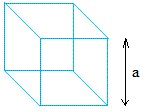Volume   = a3  = a × a × a Cylinder -: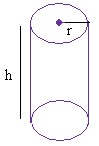Volume = pi × r2 × h pi = 3.14 h is the height r is the radius Rectangular Solid -: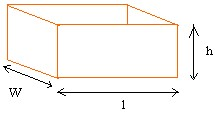Volume = l × w × h  l is the length w is the width h is the height Sphere -: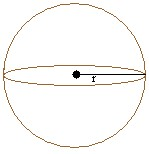Volume = (4 × pi × r3)/3  pi = 3.14 r is the radius Cone -: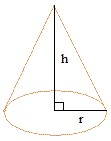Volume = (pi × r2 × h)/3 pi = 3.14 r is the radius h is the height Pyramid -: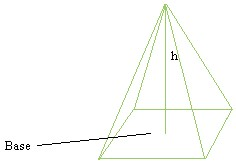Volume = (B × h)/3 B is the area of the base h is the heightRRB ALP Study notes – Sample Questions

RRB ALP Study notes – Sample Question 1

Q) A solid cylinder of brass 9 m high and 4 m in diameter is melted and recast into a cone of diameter 3 m. Find the height of the cone.

(a) 45 m

(b) 48 m

(c) 51 m

(d) 42 m

Answer -: Option (b) – 48 m

Given that, height of cylinder (h) = 9 m    and    diameter of cylinder = 4 m

∴ radius of cylinder (r) = 2 m

And, diameter of cone = 3 m

∴ radius of cone (R) = 1.5 m

Let height of Cone = H

Note: When an object is melted and given a different shape, the volumes of both the objects (of two different shapes) remain equal.

Volume of cylinder = Volume of cone

∴ πr²h = 1/3 × πR²H

⇒ (2) ² × 9 = 1/3 × (1.5) ² × H

∴ H = 36 × 3/2.25 = 48 mRRB ALP Study notes – Sample Question 2

Q) If the radius of a sphere is increased by 10% then the volume will be increased by

(a) 10%

(b) 25%

(c) 33.1%

(d) 40%

Answer -: Option (c) – 33.1%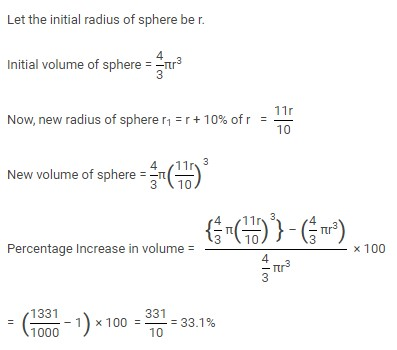RRB ALP Study notes – Sample Question 3

Q) A hollow sphere of internal and external diameters 4 cm and 8 cm respectively is melted into a cone of base diameter 8 cm. What is the height of the cone?

(a) 10 cm

(b) 25 cm

(c) 21 cm

(d) 14 cm

Answer -: Option (d) – 14

Given, internal diameter of hollow sphere = 4 cm, then internal radius (r) = 2 cm

And, external diameter of hollow sphere = 8 cm, then external radus (R) = 4 cm

Diameter of cone = 8 cm, then radius of cone (r1) = 4 cm

Let height of cone = h

Note: When an object is melted and given a different shape, the volumes of both the objects (of two different shapes) remain equal.

∴  Volume of cone = Volume of hollow sphereIf any have any further queries or suggestions regarding RRB ALP Recruitment and Group D Exam you can connect with us on FBTwitterInstagram and YouTube as well.

All the best for RRB ALP Recruitment and Group D Exam

Related Articles:

Read more Railway RRB ALP Recruitment related articles.

Interact with your peers on Railways in our discussion forum.